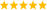# LabVIEW

cancel
Showing results for
Did you mean:

Solved!
Go to solution
Highlighted

## Superimpose a point on a graph

Hi,

I am a beginner at Labview. Attached below is the program and the Output of my Program. I have plotted the original 2 Dimensional Array of Force(Y) vs Angle(X) on the XY graph( Blue Lines and Dots) The Black Curve is the polynomial fit of this data. Now with a Peak Detector I have found the amplitude and the location of the peak (Black Square) which gives me the Side Angle and the Force. But now heres my problem, I want to find the original Force from the original data with respect to the new Side angle(X value) that I get from the Peak detector. That means, I want to superimpose this point (X Value) on the original Graph and find the respective Force (Y Value).

Can anyone tell me how I can do that?

Message 1 of 5
(892 Views)
Solution
Accepted by topic author rvkamat

## Re: Superimpose a point on a graph

Hi rvkamat,

you need to use the same Interpolate1DArray function you used to find that point on the original measurement data&colon;

Find the (broken) index in the X array and use that index to interpolate the Y data array…Best regards,
GerdW
CLAD expired, using LV2011SP1 + LV2017 (+LV2019 sometimes) on Win7+cRIO
Kudos are welcomeMessage 2 of 5
(878 Views)

## Re: Superimpose a point on a graph

Somehow the picture vanished, so let's try again:Best regards,
GerdW
CLAD expired, using LV2011SP1 + LV2017 (+LV2019 sometimes) on Win7+cRIO
Kudos are welcomeMessage 3 of 5
(866 Views)

## Re: Superimpose a point on a graph

Hi GerdW

Thanks that worked! I was trying with the Interpolate 1D function before but now it worked properly together with the Threshold 1D Function.

Message 4 of 5
(864 Views)

## Re: Superimpose a point on a graph

Alternatively, interpolate array works directly on an array of XY points, eliminating the second function. Same difference.

(Note that the points need to be sorted in X)(function is the lower part are "index & Bundle cluster array", and "interpolate array")

You can even swap the inputs to "index&Bundle ..." and then use "threshold array" instead in the same way. 😉LabVIEW Champion. It all comes together inGCentralMessage 5 of 5
(839 Views)